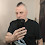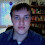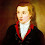## Tuesday, June 29, 2010

### From List Comprehensions to Generator Expressions

List comprehensions were added in Python 2.0. This feature originated as a set of patches by Greg Ewing with contributions by Skip Montanaro and Thomas Wouters. (IIRC Tim Peters also strongly endorsed the idea.) Essentially, they are a Pythonic interpretation of a well-known notation for sets used by mathematicians. For example, it is commonly understood that this:
{x | x > 10}

refers to the set of all x such that x > 10. In math, this form implies a universal set that is understood by the reader (for example, the set of all reals, or the set of all integers, depending on the context). In Python, there is no concept of a universal set, and in Python 2.0, there were no sets. (Sets are an interesting story, of which more in a future blog post.)

This and other considerations led to the following notation in Python:
[f(x) for x in S if P(x)]

This produces a list containing the values of the sequence S selected by the predicate P and mapped by the function f. The if-clause is optional, and multiple for-clauses may be present, each with their own optional if-clause, to represent nested loops (the latter feature is rarely used though, since it typically maps a multi-dimensional entity to a one-dimensional list).

List comprehensions provide an alternative to using the built-in map() and filter() functions. map(f, S) is equivalent to [f(x) for x in S] while filter(P, S) is equivalent to [x for x in S if P(x)]. One would think that list comprehensions have little to recommend themselves over the seemingly more compact map() and filter() notations. However, the picture changes if one looks at a more realistic example. Suppose we want to add 1 to the elements of a list, producing a new list. The list comprehension solution is [x+1 for x in S]. The solution using map() is map(lambda x: x+1, S). The part “lambda x: x+1” is Python’s notation for an anonymous function defined in-line.

It has been argued that the real problem here is that Python’s lambda notation is too verbose, and that a more concise notation for anonymous functions would make map() more attractive. Personally, I disagree—I find the list comprehension notation much easier to read than the functional notation, especially as the complexity of the expression to be mapped increases. In addition, the list comprehension executes much faster than the solution using map and lambda. This is because calling a lambda function creates a new stack frame while the expression in the list comprehension is evaluated without creating a new stack frame.

Given the success of list comprehensions, and enabled by the invention of generators (of which more in a future episode), Python 2.4 added a similar notation that represents a sequence of results without turning it into a concrete list. The new feature is called a “generator expression”. For example:
sum(x**2 for x in range(1, 11))

This calls the built-in function sum() with as its argument a generator expression that yields the squares of the numbers from 1 through 10 inclusive. The sum() function adds up the values in its argument resulting in an answer of 385. The advantage over sum([x**2 for x in range(1, 11)]) should be obvious. The latter creates a list containing all the squares, which is then iterated over once before it is thrown away. For large collections these savings in memory usage are an important consideration.

I should add that the differences between list comprehensions and generator expressions are fairly subtle. For example, in Python 2, this is a valid list comprehension:
[x**2 for x in 1, 2, 3]

However this is not a valid generator expression:
(x**2 for x in 1, 2, 3)

We can fix it by adding parentheses around the "1, 2, 3" part:
(x**2 for x in (1, 2, 3))

In Python 3, you also have to use these parentheses for the list comprehension:
[x**2 for x in (1, 2, 3)]

However, in a "regular" or "explicit" for-loop, you can still omit them:
for x in 1, 2, 3: print(x**2)

Why the differences, and why the changes to a more restrictive list comprehension in Python 3? The factors affecting the design were backwards compatibility, avoiding ambiguity, the desire for equivalence, and evolution of the language. Originally, Python (before it even had a version :-) only had the explicit for-loop. There is no ambiguity here for the part that comes after 'in': it is always followed by a colon. Therefore, I figured that if you wanted to loop over a bunch of known values, you shouldn't be bothered with having to put parentheses around them. This also reminded me of Algol-60, where you can write:
for i := 1, 2, 3 do Statement

except that in Algol-60 you can also replace each expression with step-until clause, like this:
for i := 1 step 1 until 10, 12 step 2 until 50, 55 step 5 until 100 do Statement

(In retrospect it would have been cool if Python for-loops had the ability to iterate over multiple sequences as well. Alas...)

When we added list comprehensions in Python 2.0, the same reasoning applied: the sequence expression could only be followed by a close bracket ']' or by a 'for' or 'if' keyword. And it was good.

But when we added generator expressions in Python 2.4, we ran into a problem with ambiguity: the parentheses around a generator expression are not technically part of the generator expression syntax. For example, in this example:
sum(x**2 for x in range(10))

the outer parentheses are part of the call to sum(), and a "bare" generator expression occurs as the first argument. So in theory there would be two interpretations for something like this:
sum(x**2 for x in a, b)

This could either be intended as:
sum(x**2 for x in (a, b))

or as:
sum((x**2 for x in a), b)

After a lot of hemming and hawing (IIRC) we decided not to guess in this case, and the generator comprehension was required to have a single expression (evaluating to an iterable, of course) after its 'in' keyword. But at the time we didn't want to break existing code using the (already hugely popular) list comprehensions.

Then when we were designing Python 3, we decided that we wanted the list comprehension:
[f(x) for x in S if P(x)]

to be fully equivalent to the following expansion using the built-in list() function applied to a generator expression:
list(f(x) for x in S if P(x))

Thus we decided to use the slightly more restrictive syntax of generator expressions for list comprehensions as well.

We also made another change in Python 3, to improve equivalence between list comprehensions and generator expressions. In Python 2, the list comprehension "leaks" the loop control variable into the surrounding scope:
x = 'before'
a = [x for x in 1, 2, 3]
print x # this prints '3', not 'before'

This was an artifact of the original implementation of list comprehensions; it was one of Python's "dirty little secrets" for years. It started out as an intentional compromise to make list comprehensions blindingly fast, and while it was not a common pitfall for beginners, it definitely stung people occasionally. For generator expressions we could not do this. Generator expressions are implemented using generators, whose execution requires a separate execution frame. Thus, generator expressions (especially if they iterate over a short sequence) were less efficient than list comprehensions.

However, in Python 3, we decided to fix the "dirty little secret" of list comprehensions by using the same implementation strategy as for generator expressions. Thus, in Python 3, the above example (after modification to use print(x) :-) will print 'before', proving that the 'x' in the list comprehension temporarily shadows but does not override the 'x' in the surrounding scope.

And before you start worrying about list comprehensions becoming slow in Python 3: thanks to the enormous implementation effort that went into Python 3 to speed things up in general, both list comprehensions and generator expressions in Python 3 are actually faster than they were in Python 2! (And there is no longer a speed difference between the two.)

UPDATE: Of course, I forgot to mention that Python 3 also supports set comprehensions and dictionary comprehensions. These are straightforward extensions of the list comprehension idea.

1.Very interesting and refreshing. Thanks!

2.very much informative! thanks

3.The parallel with set theory is even closer than you suggest, Guido. If you could write in set theory simply:

{ x | x > 10 }

Then that would expose you to Russell's Paradox, and you could also write:

{ x | x ∉ x }

And that would be very naughty indeed. :-)

So in real set notation, one must be so clean as to write, e.g.:

{ x ∈ ℚ | x > 10 }

Which is, after all, the same as Python list/generator comprehensions (other than a slight spelling difference).

4.Correct me if I'm wrong, but wouldn't you be able to support map/reduce just as efficiently as list comprehensions/generator expressions if you simply re-used the stack frame (a la tail recursion)?

5.@David Mertz: Very clever, but I *did* say that there was a universal set implied by the context. The math books on my shelves show lots of examples where the universal set is omitted from the notation. Also, {x ∈ ℚ | x > 10} differs from list comprehensions because the latter have to repeat "x" twice: [x for x in Q if x > 10].

@James Brown: list comprehensions *are* map/filter. As for reduce and tail recursion, that discussion is closed.

6.Are you sure about your performance figures at the end there? Comprehensions are still much faster than generator expressions in Py3k for me (which makes sense, since the comprehensions still do everything inline in one function, while the generator expression has to keep popping in and out of the generator frame from C code). To avoid the name lookup confounding the relative timings, I used the following timeit snippets:

./python -m timeit -s "seq = *1000" "[x for x in seq]; list"
./python -m timeit -s "seq = *1000" "{x for x in seq}; list
python -m timeit -s "seq = *1000" "list(x for x in seq)"

Those timings were in the vicinity of 45-50 us, 60-70 us, 75-85 us.

The raw speed of the operations is also pretty similar between 2.7 and 3.2 for me (neither being noticeably slower or faster than the other just eyeballing the timeit results). Although both seem a little faster than 2.6, so maybe the speedups were backported along with dict and set comprehensions (which seems likely, since it should be the same code that handles it all in both branches now).

One thing that *is* much faster in Py3k is a module level list comprehension, since those now automatically benefit from function local variable access optimisations for their loop variables.

7.Oops, there should be a "./" at the start of last timeit snippet as well (and there was in the shell where I was running the test).

8.@Nick: Thanks for the detailed timings. I had timed something similar for a much smaller sequence, so my numbers probably include more per-loop setup overhead.

9.@James Brown:

It can be difficult to determine what qualifies as an implementation detail and what qualifies as a language feature, especially in the absence of a standard. While I think we have a great understanding of where that line lies with Python today, there is one subject where the distinction is fairly clear, that is, when talking about speed. If we are talking about the time order of an operation, then it is possibly part of the language (list indexing is specified as an O(1) operation in python). Otherwise, the time taken for any expression to execute is obviously not considered part of the language; as long as evaluation of some expression terminates, it can take as long as it likes.

10.It seems like it shouldn't be bad for generator expressions to be a small constant factor slower than list comprehensions. Aren't generator expressions primarily a memory optimization, and only secondarily possibly a speed optimization? I guess it's bad if you write "sum(x*x for x in L)" and it's slower than "sum([x*x for x in L])"...